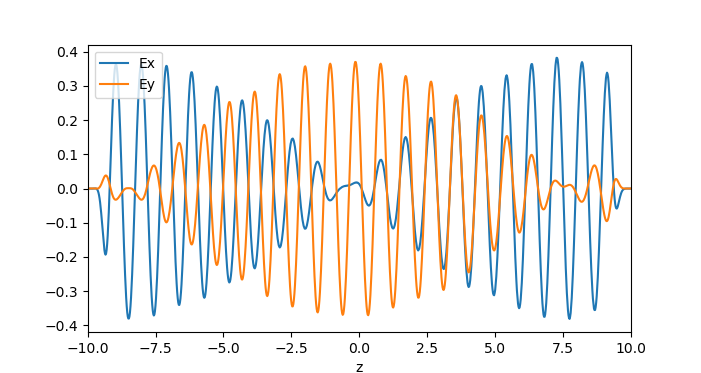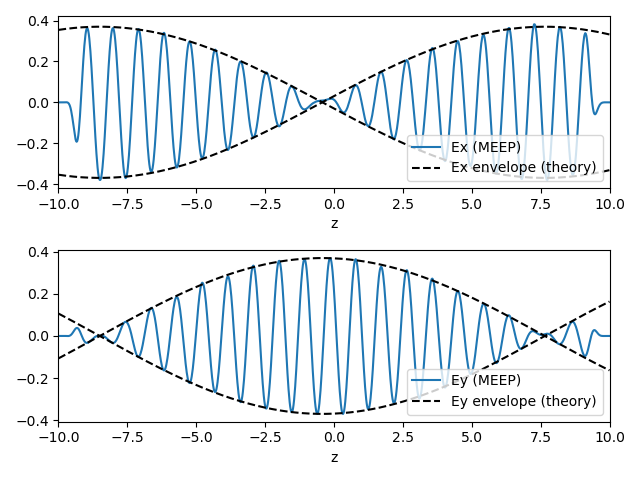# Gyrotropic Media

In this example, we will perform simulations with gyrotropic media. See Materials for more information on how gyrotropy is supported.

Consider a uniform gyroelectric medium with bias vector $\mathbf{b} = b \hat{z}$. In the frequency domain, the x and y components of the dielectric tensor have the form

The skew-symmetric off-diagonal components give rise to Faraday rotation: when a plane wave linearly polarized along x is launched along the gyrotropy axis z, the polarization vector will precess around the gyrotropy axis as the wave propagates. This is the principle behind Faraday rotators, devices that act as one-way valves for light.

A plane wave undergoing Faraday rotation can be described by the complex ansatz

where $\kappa_c$ is the Faraday rotation (in radians) per unit of propagation distance. Substituting this into the frequency domain Maxwell's equations, with the above dielectric tensor, yields

We model this phenomenon in the simulation script faraday-rotation.ctl. First, we define a gyroelectric material:

(define-param epsn 1.5)    ; background permittivity
(define-param f0 1.0)      ; natural frequency
(define-param g0 1e-6)     ; damping rate
(define-param sn 0.1)      ; sigma parameter
(define-param b0 0.15)     ; magnitude of bias vector

(set! default-material
(make dielectric
(epsilon epsn)
(E-susceptibilities
(make gyrotropic-lorentzian-susceptibility
(frequency f0)
(sigma sn)
(gamma g0)
(bias (vector3 0 0 b0))))))


The gyrotropic-lorentzian-susceptibility object has a bias argument that takes a vector3 specifying the gyrotropy vector. In this case, the vector points along z, and its magnitude (which specifies the precession frequency) is determined by the variable b0. The other arguments play the same role as in an ordinary (non-gyrotropic) Lorentzian susceptibility.

Next, we set up and run the Meep simulation.

(define-param tmax 100)
(define-param L 20.0)
(define-param fsrc 0.8)
(define-param src-z -8.5)
(set-param! resolution 50)

(set! geometry-lattice (make lattice (size 0 0 L)))

(set! pml-layers (list (make pml (thickness 1.0) (direction Z))))

(set! sources (list
(make source
(src (make continuous-src (frequency fsrc)))
(component Ex)
(center (vector3 0 0 src-z)))))

(run-until tmax
(to-appended "efields"
(at-end output-efield-x)
(at-end output-efield-y)))


The simulation cell is one pixel wide in the x and y directions, with periodic boundary conditions. PMLs are placed in the z direction. A ContinuousSource emits a wave whose electric field is initially polarized along x.

After running the simulation, the ex and ey datasets in faraday-rotation-efields.h5 contain the values of $\mathbf{E}_x$ and $\mathbf{E}_y$. These are plotted against z in the figure below:We see that the wave indeed rotates in the x-y plane as it travels.

Moreover, we can compare the Faraday rotation rate in these simulation results to theoretical predictions. In the gyrotropic Lorentzian model, the ε tensor components are given by

From these expressions, we can calculate the rotation rate $\kappa_c$ at the operating frequency, and hence find the $\mathbf{E}_x$ and $\mathbf{E}_y$ field envelopes for the complex ansatz given at the top of this section. As shown in the figure below, the results are in excellent agreement: